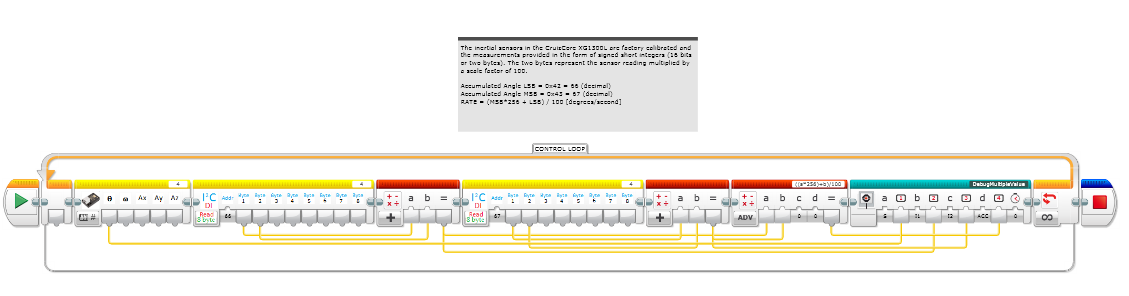# How to read a short integer stored in 2 bytes with the block I2C Read 8 Bytes?

Hi,

last week, I learnt how to read values from I2C for registers which It returns 1 byte of information (0-128) but now, reading again the technical documentation, I read this fact:

“the measurements provided in the form of signed short integers (16 bits or two bytes).”In the example I sum both values from byte 1 and byte 2, but maybe it is not the right operation.

So, how to show a integer in the register 0x43, 66 with the block I2C Read 8 Bytes?

If I get the first byte and the second one, how to show the right signed short integer?

Cheers

Juan Antonio

Hi Juan,

Each byte should be 8 bits (0-255). If a 16-bit integer is stored/transferred using two bytes, then to “unpack” the 2 x 8 bits into 16 bits, you would do something like:

``````value = (byte_low | (byte_high << 8))
``````

I don’t know if the EV3 software allows you to use bitwise math. It’s probably easier to do something like:

``````value = (byte_low + (byte_high * 256))
``````

If value needs to end up being a 16-bit signed value (as opposed to unsigned), then in C you could type cast it to a int16_t, but in EV3 software you would probably need to do something like:

``````if value > 32767
value = value - ‭65536
``````

Which byte is high and which is low is dependent on the device you’re trying to read from. The device datasheet should tell you how the registers are configured.

Matt

Hi Matt,

I am going to test now!

Thanks mate